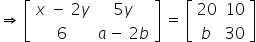Maths-
General
Easy

Question

# Ifthen a + x =

## 42    24    60    66Hint:

## The correct answer is: 66

### Given :Equating corresponding elements, we getx - 2y = 205y = 10 , y = 2Substitute they value of y in x - 2y = 20 and find xx - 2(2) = 20x = 24b = 6a- 2b = 30a - 2(6) = 30a = 42a + x = 42 + 24 = 66#### With Turito Foundation.#### Get an Expert Advice From Turito.